# Python Plotting With Pyplot MCQ | Python Data VisualizationThis post contains Multiple Choice based Questions with answers for Python Plotting With Pyplot MCQ. These objective questions with answers are not only helpful for those students preparing for CBSE IP Class 12 Python Plotting With Pyplot MCQ but also for all those students who are practicing and learning Python Programming specially Data Handling and Visualization using Python Matplotlib Plotting with pyplot .

You will get different categories of Questions for all the concept of Python Matplotlib Plotting with pyplot objective questions-

Assertion and Reason MCQs
Case Study Based MCQs
Find The Output Based MCQs
Concept Based MCQs

#### Python Plotting With Pyplot MCQ SET-2 (Q26-Q50)

1. box plot is visual representation of statistical ______ summary of given dataset
a. Three number
b. Five number
c. Seven number
d. Nine number

b. Five Number

1. To import pyplot module we can write

a. Import pyplot as plt
b. Import matplotlib.pyplot
c. Import matplotlib.pyplot as plt
d. Both a and c
e. Both b and c

e. Both b and c

1. Chart given below is type of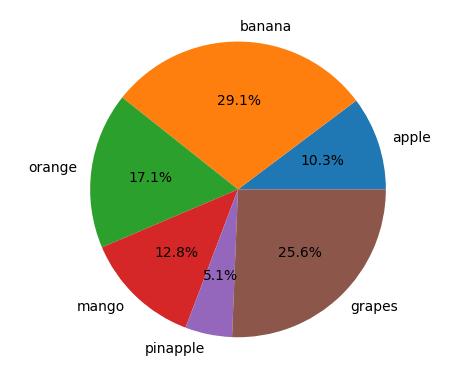a. Area Chart
b. Pie Chart
c. Surface Chart
d. Bubble Chart

b. Pie Chart

1. To create a chart, pyplot provides

a. figure()
b. chart()
c. plot()
d. print()

c. plot()

1. Which of the following type of chart is not valid?

a. Line
b. Pie
c. Curve
D. Bar

c. Curve

1. to create histogram pyplot provides

a. hist()
b. histo()
c. histogram()
d. histg()

a. hist()

1. Look at the following graph and select appropriate code to obtain this output. (Please assume that pandas and matplotlib is already imported)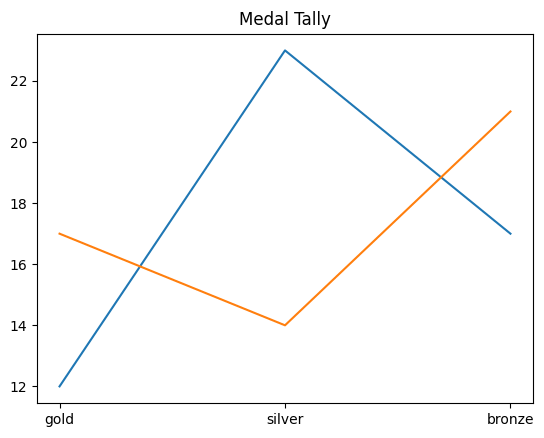a. medal = [‘gold’,’silver’,’bronze’]
delhi = [12,23,17]
mumbai = [17,14,21]
plt.plot(medal,delhi)
plt.plot(medal,mumbai)
plt.title(“Medal Tally”)
plt.show()

b. df = d.DataFrame({‘Delhi’:[12,23,17],’Mumbai’:[17,14,21]},index=[‘gold’,’silver’,’bronze’])
df.plot(kind = ‘line’)
plt.title(“Medal Tally”)
plt.show()

c. df = d.DataFrame({‘Delhi’:[12,23,17],’Mumbai’:[17,14,21]},index=[‘gold’,’silver’,’bronze’])
df.plot(kind = ‘line’, ”Medal Tally”)
plt.show()

d. a and b
e. a and c

d. a and b

33. Which of the following option describes a pie chart?

a. Comparison in different categories of data
b. Relationship between two sets of numeric data
c. Contribution of individual values to a total value
d. Data trend over a period of time

c. Contribution of individual values to a total value

34. We can display more than one data series together in

a. Line
b. Bar
c. Pie
d. Both a and b
e. All of the above

d. Both a and b

35. Which of the following is best suitable chart to represent continuous data?

a. Bar
b. Line
c. Scatter
d. Histogram

d. Histogram

36. Consider the following code written to display a bar chart

X=range(0,40,8)
Y=range(10,100,10)
Plt.bar(X,Y)

While executing it, is producing error. Why?

a. Both the sequences X and Y do not start with 0
b. Both the sequence X and Y are not of same shape
c. Values produced by range is not considered for chart
d. Both the sequences X and Y have values with different intervals

b. Both the sequence X and Y are not of same shape

37. to create column chart we can use

a. bar()
b. barh()
c. box()
d. plot()

a. bar()

38. To view changes in data over a period of time we can use ________ chart.

a. Line
b. Bar
c. Histogram
d. Pie

a. Line

39. Which shape of marker will be printed in the scatter chart made using following code
Plt.scatter(x,y,marker = ‘D’)

a. Down triangle
b. Diamond
c. Double circle
d. Donut

b. Diamond

40 .Identify the following chart type.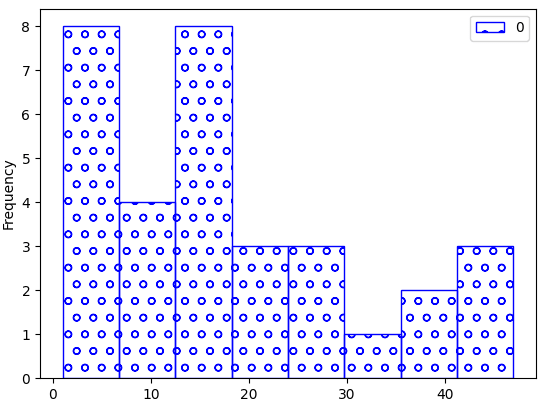a. Bar Chart
b. Histogram
c. Frequency Polygon
d. Box Plot

b. Histogram

41. which of the following is not a type of bar chart?

a. Column chart
b. Bar chart
c. Histogram
d. Scatter chart

d. Scatter chart

42. Which of the following is correct syntax for displaying title on chart?

a. Plt.title = “My Title”
b. Plt.title()=”My Title”
c. Plt.title[“My Title”]
d. Plt.title(“My Title”)

d. Plt.title(“My Title”)

43. In histogram, it describes the no of data points that falls within a range of given values

a. bin
b. bins
c. range
d. range()

b. bins

44. in the given chart, box surrounded with red color is called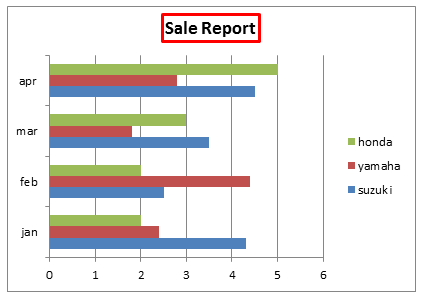a. Data series
b. Chart title
c. Markers
d. Legend

b. Chart title

45. The best suitable chart to display data trend is

a. Line
b. Bar
c. Pie
d. Histogram

a. Line

46. The difference between histogram and bar chart is
(i). Bar chart is used to represent continuous values and histogram represent discrete values
(ii). There is no gap between bars in histogram however in bar chart gap exists.

a. (i)  True, (ii) False
b. (i) False, (ii) True
c. Both are True
d. Both are False

b. (i) False, (ii) True

47. Which statement is not true about scatter chart?

a. Also known as correlation chart
b. It is primarily used to represent relation between two sets of data
c. The closer the dots to line, the weaker the relation will be
d. It uses dots to represent data points

c. The closer the dots to line, the weaker the relation will be

48. A chart represent the _________ between different elements of data categories

a. Relationship
b. Difference
c. Variance
d. Similarity

a. Relationship

49. Graph which can be plotted vertically or horizontally is

a. Bar
b. Scatter
c. Line
d. Pie

a. Bar

50. in the given chart, points surrounded with circle is called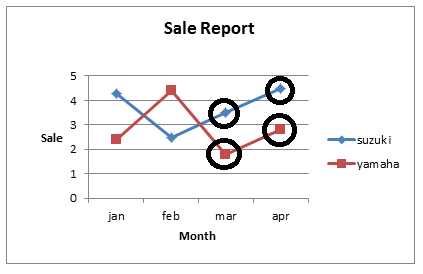a. Label
b. Ticks
c. Markers
d. Series

c. Markers

### 2 thoughts on “Python Plotting With Pyplot MCQ | Python Data Visualization”

1.great

error: Content is protected !!